Processing ......FreeComputerBooks.com Free Computer, Mathematics, Technical Books and Lecture Notes, etc.

Data Science
Related Book Categories:
 Data Analysis and Data MiningBig Data Python Programming Non-relational/NoSQL Databases Statistics and SAS Programming Database Theory and Systems Machine LearningDeep Learning & Neural Networks R Programming Information Retrieval & Search Engines Data Tools Research Library SQL - The Structured Query Language
•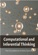Computational and Inferential: The Foundations of Data Science

Step by step, you'll learn how to leverage algorithmic thinking and the power of code, gain intuition about the power and limitations of current machine learning methods, and effectively apply them to real business problems.

•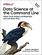Data Science at the Command Line (Jeroen Janssens)

This hands-on guide demonstrates how the flexibility of the command line can help you become a more efficient and productive data scientist. You'll learn how to combine small, yet powerful, command-line tools to quickly obtain, scrub, explore, and model your data.

•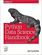O'Reilly® Python Data Science Handbook: Essential Tools

Several resources exist for individual pieces of this data science stack, but only with the Python Data Science Handbook do you get them all - IPython, NumPy, Pandas, Matplotlib, Scikit-Learn, and other related tools.

•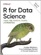R for Data Science: Visualize, Model, Transform, Tidy, & Import

This book teaches you how to do data science with R: You'll learn how to get your data into R, get it into the most useful structure, transform it, visualize it and model it, how data science can help you work with the uncertainty and capture the opportunities.

•The Data Science Handbook: Advice and Insights

This book covers the essential exploratory techniques for summarizing data with R. These techniques are typically applied before formal modeling commences and can help inform the development of more complex statistical models.

•Exploring Data Science (Nina Zumel, et al)

This book introduces readers to various areas in data science and explains which methodologies work best for each, with practical examples in R, Python, and other languages.

•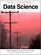Introduction to Data Science (Jeffrey Stanton)

This book provides non-technical readers with a gentle introduction to essential concepts and activities of data science. For more technical readers, the book provides explanations and code for a range of interesting applications using the open source R language for statistical computing and graphics.

•Data Assimilation: A Mathematical Introduction (Kody Law, et al)

This book provides a systematic treatment of the mathematical underpinnings of work in data assimilation, covering both theoretical and computational approaches. Specifically the authors develop a unified mathematical framework of Bayesian formulation.

•School of Data Handbook

This textbook will provide the detail and background theory to support the Data Science courses and challenges. It will guide you through the key stages of a data project. These stages can be thought of as a pipeline, or a process.

•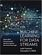Machine Learning for Data Streams: Practical Examples in MOA

This book presents algorithms and techniques used in data stream mining and real-time analytics. Taking a hands-on approach, it demonstrates the techniques using MOA (Massive Online Analysis), a popular, freely available open-source software framework, allowing readers to try out the techniques after reading the explanations.

•Big Data Processing with Apache Spark (Srini Penchikala)

Learn about the Apache Spark framework and develop Spark programs for use cases in big-data analysis. It covers all the libraries that are part of Spark ecosystem, which includes Spark Core, Spark SQL, Spark Streaming, Spark MLlib, and Spark GraphX.

•Hadoop with Python (Zachary Radtka, et al)

This book takes you through the basic concepts behind Hadoop, MapReduce, Pig, and Spark. Then, through multiple examples and use cases, you'll learn how to work with these technologies by applying various Python tools.

•Mastering Apache Spark 2.0 (Jacek Laskowski)

This book introduces Apache Spark, the open source cluster computing system that makes data analytics fast to write and fast to run. With Spark, you can tackle big datasets quickly through simple APIs in Python, Java, and Scala.

•The Fourth Paradigm: Data-Intensive Scientific Discovery

This book presents the first broad look at the rapidly emerging field of data-intensive science, with the goal of influencing the worldwide scientific and computing research communities and inspiring the next generation of scientists.

•Modeling with Data: Tools and Techniques for Scientific Computing

Modeling with Data fully explains how to execute computationally intensive analyses on very large data sets, showing readers how to determine the best methods for solving a variety of different problems, etc..

•Exploring Data in Python 3

This book is designed to introduce students to programming and software development through the lens of exploring data. You can think of the Python programming language as your tool to solve data problems that are beyond the capability of a spreadsheet.

•Think Stats, 2nd Edition: Exploratory Data Analysis in Python

This concise introduction shows you how to perform statistical analysis computationally, rather than mathematically, with programs written in Python.

•O'Reilly® Think Bayes: Bayesian Statistics Made Simple

An introduction to Bayesian statistics using computational methods and Python. You'll learn how to solve statistical problems with Python code instead of mathematical notation, and use discrete probability distributions instead of continuous mathematics.

•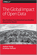The Global Impact of Open Data: Key Findings from Case Studies

Open data has spurred economic innovation, social transformation, etc. This book presents detailed case studies of open data projects throughout the world, along with in-depth analysis of what works and what doesn't.

•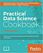Practical Data Science Cookbook (Prabhanjan Tattar, et al)

Starting with the basics, this book covers how to set up your numerical programming environment, introduces you to the data science pipeline, and guides you through several data projects in a step-by-step format.

•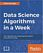Data Science Algorithms in a Week: Top 7 Algorithms

This book will address the problems related to accurate and efficient data classification and prediction. Over the course of 7 days, you will be introduced to seven algorithms, along with exercises that will help you learn different aspects of machine learning.

Book CategoriesAll CategoriesRecent BooksIT Research LibraryMiscellaneous Books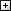Computer LanguagesComputer ScienceData Science/DatabasesElectronic EngineeringJava and Java EE (J2EE)Linux and UnixMathematicsMicrosoft and .NETMobile ComputingNetworking and CommunicationsSoftware EngineeringSpecial TopicsWeb Programming
Other Categories